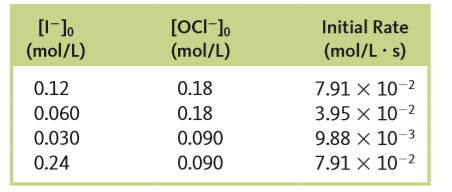# Problem: The reactionI - (aq) + OCl - (aq) → IO - (aq) + Cl - (aq)was studied, and the following data were obtained:a. What is the rate law?

###### FREE Expert Solution

We’re being asked to calculate the rate law based on the reaction and data given.

I - (aq) + OCl - (aq) → IO - (aq) + Cl - (aq)

Recall that the rate law only focuses on the reactant concentrations and has a general form of:

k = rate constant
A & B = reactants
x & y = reactant orders

We’re going to calculate the rate law and the rate constant using the following steps:

93% (127 ratings)###### Problem Details

The reaction

I - (aq) + OCl - (aq) → IO - (aq) + Cl - (aq)

was studied, and the following data were obtained:a. What is the rate law?

Frequently Asked Questions

What scientific concept do you need to know in order to solve this problem?

Our tutors have indicated that to solve this problem you will need to apply the Rate Law concept. You can view video lessons to learn Rate Law. Or if you need more Rate Law practice, you can also practice Rate Law practice problems.

What professor is this problem relevant for?

Based on our data, we think this problem is relevant for Professor Blake's class at UCI.

What textbook is this problem found in?

Our data indicates that this problem or a close variation was asked in Chemistry: An Atoms First Approach - Zumdahl Atoms 1st 2nd Edition. You can also practice Chemistry: An Atoms First Approach - Zumdahl Atoms 1st 2nd Edition practice problems.